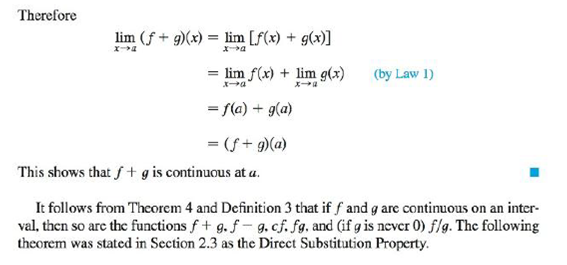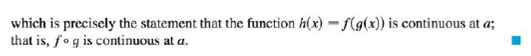Chapter 2.5, Problem 25E### Single Variable Calculus: Early Tr...

8th Edition
James Stewart
ISBN: 9781305270343

#### Solutions

Chapter
Section### Single Variable Calculus: Early Tr...

8th Edition
James Stewart
ISBN: 9781305270343
Textbook Problem

# Explain, using Theorems 4, 5, 7, and 9, why the function is continuous at every number in its domain. State the domain.Theorem 4Theorem 5Theorem 7Theorem 9F ( x ) = 2 x 2 − x − 1 x 2 + 1

To determine

To state: The domain and explain the function is continuous at every number in its domain.

Explanation

Given:

The function F(x)=2x2x1x2+1.

Theorem 5:

(a) Any polynomial is continuous everywhere; that is, it is continuous on =(,).

(b) Any rational function is continuous wherever it is defined; that is, it is continuous on its domain.

Calculation:

The domain is the set of all input values of the function for which the function is real and defined.

Consider the denominator of the function F(x) and equate to zero to obtain the undefined points.

Since the denominator of F(x) is x2+1, the undefined points are obtained as shown below:

x2+1=0x2=1x=1x=±i

Thus, there is no solutions of x found in .

Hence, it can be concluded that there is no undefined point.

Therefore, the domain of the function is the set of all real numbers or {x|x}.

And, the interval notation of the domain of F(x) is (,)

### Still sussing out bartleby?

Check out a sample textbook solution.

See a sample solution

#### The Solution to Your Study Problems

Bartleby provides explanations to thousands of textbook problems written by our experts, many with advanced degrees!

Get Started

#### Find more solutions based on key concepts